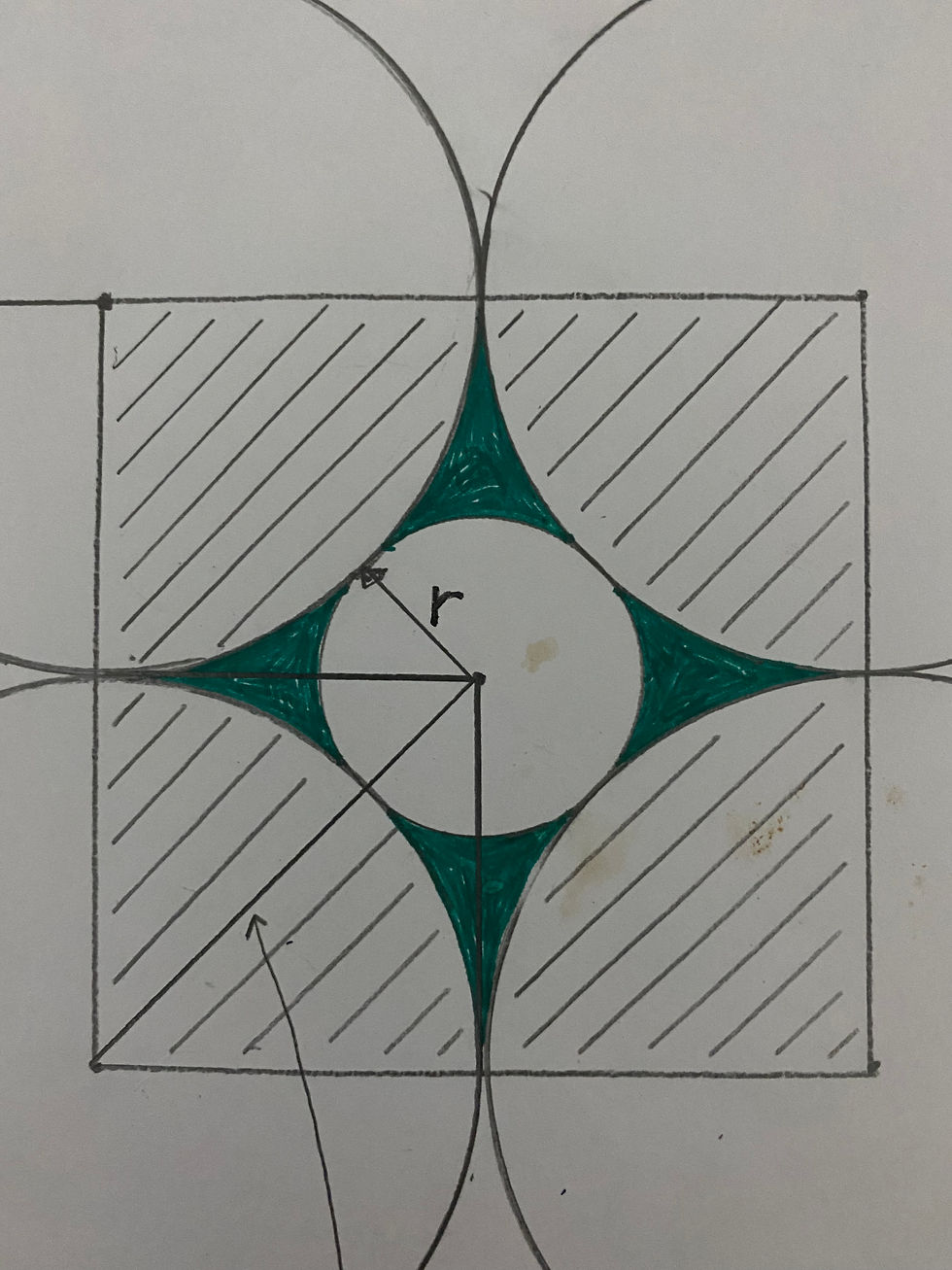top of page
Search

# Tree Puzzle Solved!

Updated: Apr 29, 2022

Within less than a day, Jim Rogala solved the Tree puzzle. Congratulations Jim! He will get the maple syrup!

He describes his methods well in the comments section accompanying the previous post, but here's a few pictures to illustrate his method.

First he constructed a square from the center of one of the big circles and drew a diagonal to the center of the little circle like this:As seen in the picture, the diagonal is R + r, which can be found by the good old Pythagorean Theorem. Remember that R = 20 from the previous post.Next, to find the left over green space, it's just the big square, from the centers of the big circles, minus one big circle from the 4 quarters seen with hatch marks, minus the little circle.Knowing that the area of a circle is Pi times the radius squared, the equation looks like this:So, the answers have been found. Again, congratulations.

But wait...that little r has to have some special meaning. A small circle embedded in four larger ones that are touching. That little r should be too good for nature to pass up. How about R divided by r? This would be 20/8.284 = 2.41429... Is that a special number?

First, from a previous post, remember the Golden Ratio could be approximated by the Fibonacci Sequence, 0, 1, 1, 2, 3, 5, 8, 13, 21, 34, 55, 89... where the subsequent number in the series is derived by adding the previous two. And that dividing one number by the previous one gives a number that is ever closer to the Golden Ratio, but never hits it exactly. So, 13/8 = 1.625, 55/34 = 1.6176, 89/55 = 1.61818, but not exactly hitting the exact ratio of 1.61803.....

Now look at this sequence: 0, 1, 2, 5, 12, 29, 70, 169,.... where each number is derived by multiplying the previous number by 2 and adding the one before that. So, "29" is derived by multiplying 12 by 2 then adding 5 to it. Next, divide one number by the previous one, just like with the Golden Ratio and Fibonacci Sequence. Let's pick 12/5 = 2.4, then 29/12 which equals 2.4166..., then 70/29 = 2.41379..., and last 109/70 = 2.41428.... Wow, that's getting closer and closer to our R divided by r above!

Last, get out your calculator and find 1 plus the square root of 2? This equals 2.4142135...! Again, very close to our number.

What is this number 2.4142135... that came from the likes of growing trees? Is this another magic ratio?

Again, the first person (board members excluded) who posts a correct answer (what is this number, how is it derived, what is its significance) in the comments section will receive a bottle of maple syrup!